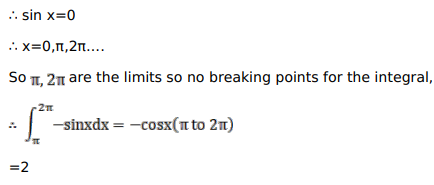# Mark against the correct answer in the following:

Question:

Mark $(\sqrt{)}$ against the correct answer in the following:

$\int_{\pi}^{2 \pi}|\sin x| d x=?$

A. 0

B. 1

C. 2

D. none of these

Solution: